CBSE Class 10 Sample Paper for 2022 Boards [Term 2] - Science Solution

Class 10
Solutions to CBSE Sample Paper - Science Class 10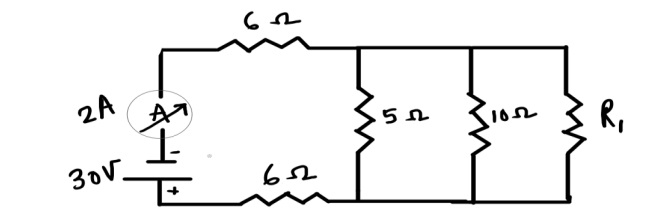## In the above circuit, if the current reading in the ammeter A is 2A, what would be the value of R 1 ?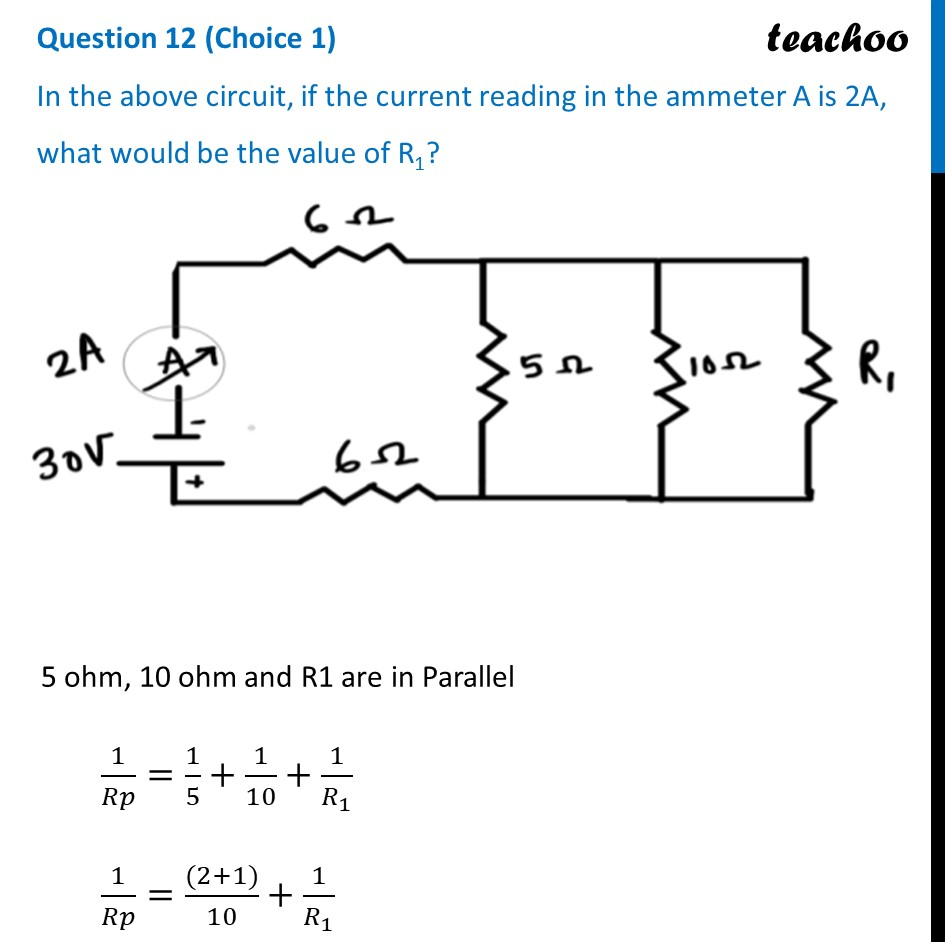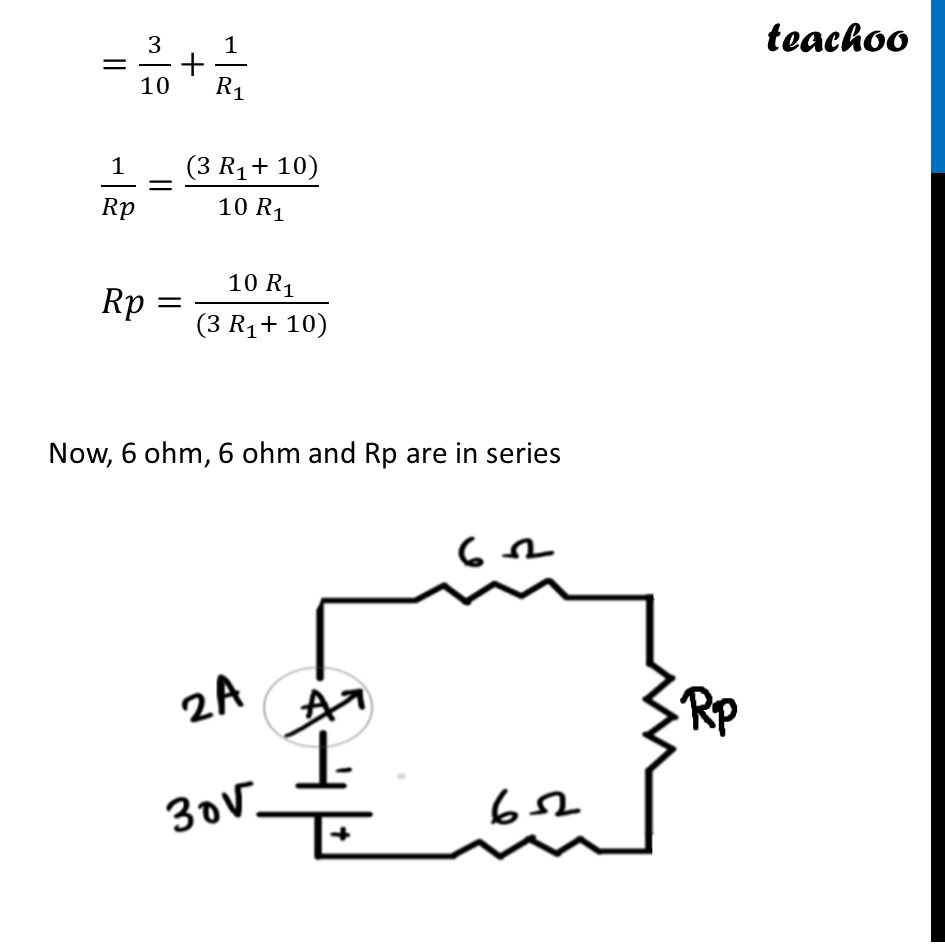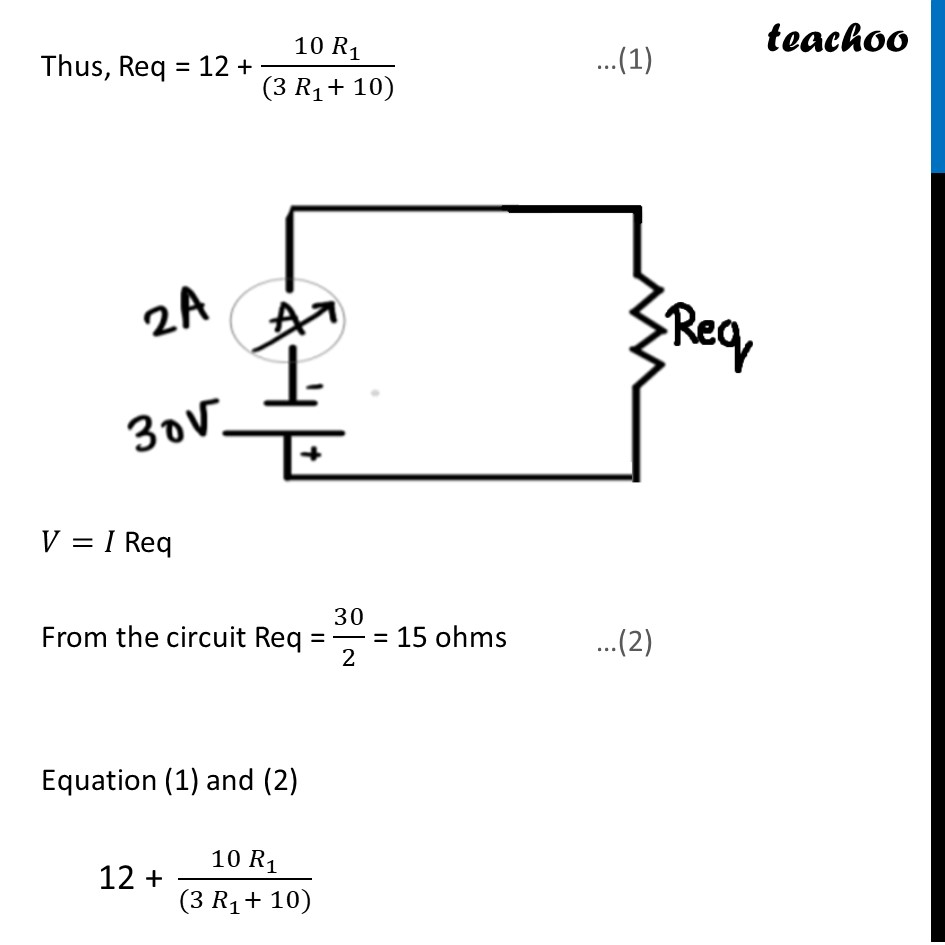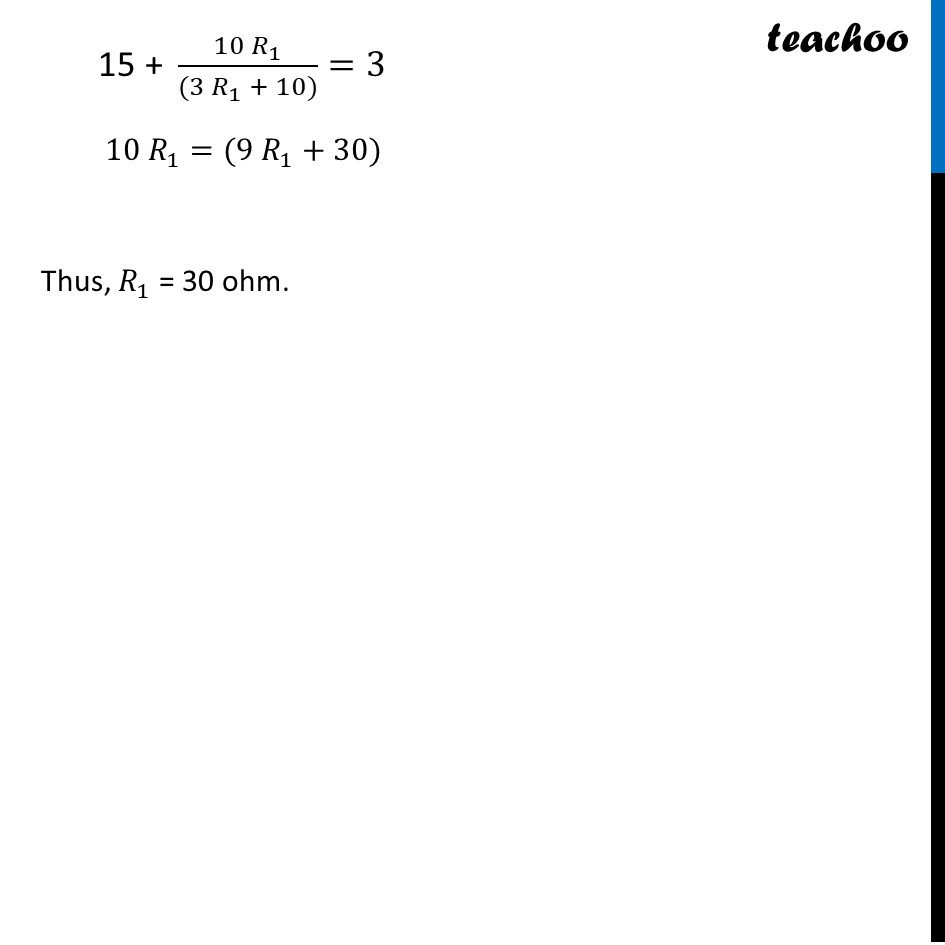Get live Maths 1-on-1 Classs - Class 6 to 12

### Transcript

Question 12 (Choice 1) In the above circuit, if the current reading in the ammeter A is 2A, what would be the value of R1? 5 ohm, 10 ohm and R1 are in Parallel 1/𝑅𝑝=1/5+1/10+1/𝑅_1 1/𝑅𝑝=((2+1))/10+1/𝑅_1 =3/10+1/𝑅_1 1/𝑅𝑝=((3 𝑅_1+ 10))/(10 𝑅_1 ) 𝑅𝑝=(10 𝑅_1)/((3 𝑅_1+ 10)) Now, 6 ohm, 6 ohm and Rp are in series Thus, Req = 12 + (10 𝑅_1)/((3 𝑅_1+ 10)) 𝑉=𝐼 Req From the circuit Req = 30/2 = 15 ohms Equation (1) and (2) "12 + " (10 𝑅_1)/((3 𝑅_1+ 10)) "15 + " (10 𝑅_1)/((3 𝑅_1 + 10))=3 10 𝑅_1=(9 𝑅_1+30) Thus, 𝑅_1 = 30 ohm.